# 一种改进变步长自适应扰动观察法的研究A Study of Improved Variable Step Size Adaptive Disturbance Observation

DOI: 10.12677/SG.2018.81003, PDF, HTML, XML, 下载: 807  浏览: 2,062

Abstract: Photovoltaic panels are effective means of solar energy utilization, and maximum power tracking is an important way to optimize this method. At present, the disturbance observing method is a widely used method of MPPT. However, the traditional disturbance observing method has the problems of tracking speed and energy fluctuation loss. In view of this, a variable step adaptive MPPT tracking method is designed. The method uses the power difference between the front and the rear moment as the duty cycle adjustment factor, and sets the threshold value, leaving a certain power fluctuation margin, which effectively solves the above existing problems; besides, it can also carry out adaptive control. By Simulink modeling and simulation analysis, compared with the traditional methods, the conclusion is drawn that the method has obvious advantages in terms of tracking stability, rapidity and energy loss reduction.

1. 引言

${g}_{k}=g\left({U}_{k}\right)=\frac{\text{d}p}{\text{d}U}$ (1)

${U}_{k+1}={U}_{k}+{a}_{k}{g}_{k}$ (2)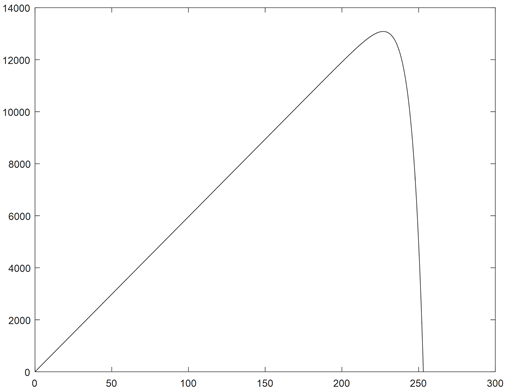Figure 1. P-U characteristic graph

2. 光伏电池建模

$I={I}_{sc}\left[1-{C}_{1}\left({\text{e}}^{\frac{U}{{C}_{2}{U}_{oc}}}-1\right)\right]$ (3)

${C}_{1}=\left(1-\frac{{I}_{m}}{{I}_{sc}}\right){\text{e}}^{\frac{-U}{{C}_{2}{U}_{oc}}}$ (4)

${C}_{2}=\left(\frac{{U}_{m}}{{U}_{oc}}-1\right){\left[\mathrm{ln}\left(1-\frac{{I}_{m}}{{I}_{sc}}\right)\right]}^{-1}$ (5)Figure 2. Photovoltaic cell equivalent model

$\Delta S=\frac{S}{{S}_{ref}}-1$ (6)

$\Delta T=T-{T}_{ref}$ (7)

${I}_{sc}^{*}={I}_{sc}\frac{S}{{S}_{ref}}\left(1+\alpha \Delta T\right)$ (8)

${U}_{oc}^{*}={U}_{oc}\left(1-\gamma \Delta T\right)\mathrm{ln}\left(e+\beta \Delta S\right)$ (9)

${I}_{m}^{*}={I}_{m}\frac{S}{{S}_{ref}}\left(1+\alpha \Delta T\right)$ (10)

${U}_{m}^{*}={U}_{m}\left(1+\gamma \Delta T\right)\mathrm{ln}\left(e+\beta \Delta S\right)$ (11)

3. 最大功率跟踪模型分析

${Z}_{in}={Z}_{L}$ (12)

${U}_{in}{I}_{s}={U}_{L}{I}_{L}$ (13)

${U}_{L}=\frac{1}{1-\alpha }{U}_{S}$ (14)

${I}_{L}=\left(1-\alpha \right){I}_{S}$ (15)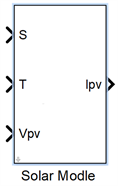Figure 3. Photovoltaic cell physics model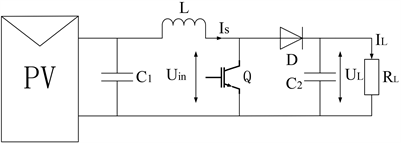Figure 4. Impedance conversion circuit

${R}_{in}=\frac{{U}_{S}}{{I}_{S}}=\frac{\left(1-\alpha \right){U}_{L}}{\frac{{I}_{L}}{1-\alpha }}={\left(1-\alpha \right)}^{2}{R}_{L}$ (16)

4. 变步长自适应扰动法原理

$\Delta D=a×|{P}_{k}-{P}_{k-1}|$ (17)

$\Delta D$ 为占空比增量，a为步长因子系数。式(17)可得，采用前后时刻功率差值作为变步长的扰动步长因子，随着功率趋近最大值的程度，差值的模也会随之改变，从而实现自适应跟踪目。

5. 仿真及数据分析

5.1. 系统∆P曲线变化分析

$\Delta P$ 曲线变化如图6所示。(a)图为改进后的功率波动图，(b)图为最优梯度法功率波动图。由于改进型方法设定了功率阈值，所以功率的波动被限制在50W以内。结合图(a)和图7中可以得出，随着光照强度的增加，系统功率在不断增加，但系统的 $\Delta P$ 也在增大，但都没有超出阈值范围。

5.2. 系统功率跟踪分析

5.2.1. 追踪速率方面

5.2.2. 最大功率方面

5.3. 占空比分析

6. 结论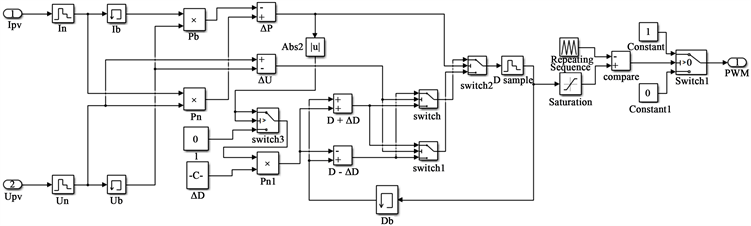Figure 5. MPPT controller schematic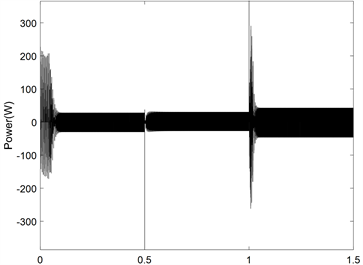(a)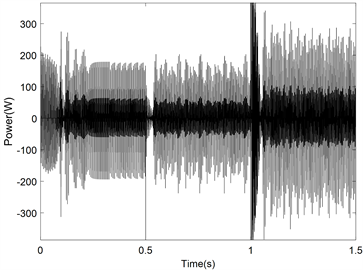(b)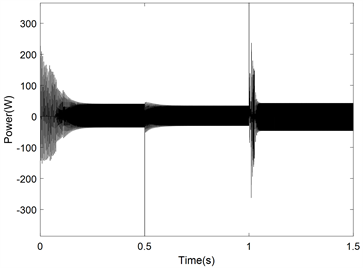(c)

Figure 6. Power fluctuation diagram. (a) Improved step-by-step adaptive method ΔP curve; (b) optimal gradient method ΔP curve; (c) set the threshold of the optimal gradient method ΔP curve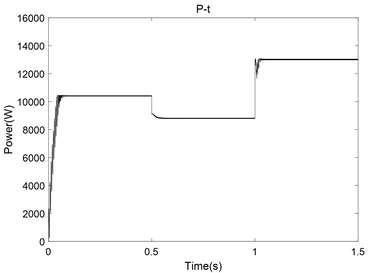(a)(b)

Figure 7. Power tracking process diagram. (a) Improved variable step adaptive tracking process diagram; (b) the best gradient method to track the process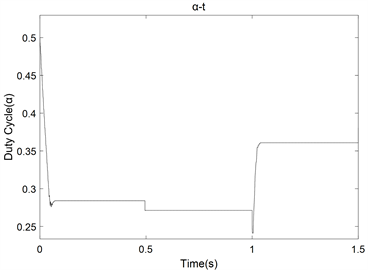(a)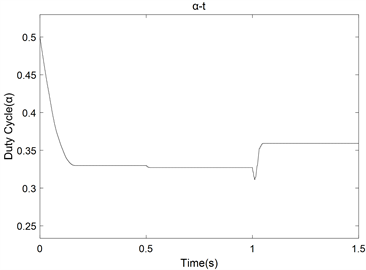(b)

Figure 8. Duty Cycle Variation Graph. (a) Improved variable step size adaptive duty cycle curve; (b) optimum gradient method duty cycle curve

  金碚. 资源与环境约束下的中国工业发展[J]. 宏观经济管理, 2004(4): 5-14.  包婧文. 光伏产业: 回顾2015展望2016[J]. 太阳能, 2016(2): 79-80.  杨立勇, 毛鹏. 光伏发电系统的优化——建模、仿真和控制[M]. 北京: 机械工业出版社, 2014.  吴大中, 王晓伟. 一种光伏MPPT模糊控制算法研究[J]. 太阳能学报, 2011, 32(6): 808-813.  朱建波, 张正江, 朱志亮, 吴平. 基于 PI 调节占空比的自适应 MPPT 方法[J]. 计算机测量与控制, 2017, 25(7): 194-197.  陈亚爱, 周京华, 李津, 周玲玲. 梯度式变步长MPPT算法在光伏系统中的应用[J]. 中国电机工程学报, 2014, 34(19): 3156-3161.  赵波. 微电网优化配置关键技术及应用[M]. 北京: 科学出版社, 2016.  邱星星, 何怡刚. 基于功率预测的最优梯度变步长MPPT算法的研究[J]. 电源技术, 2016, 40(10): 1965-1968.  张兴, 曹仁贤等. 太阳能光伏并网发电及其逆变控制[M]. 北京: 机械工业出版社, 2016.